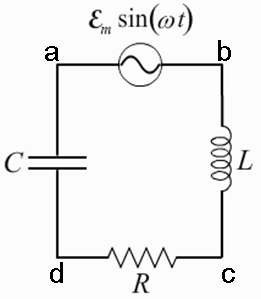# Problem: A circuit is constructed with an AC generator, a resistor, capacitor and inductor as shown. The generator voltage varies in time as ε =Va - Vb = εmsinωt, where εm = 120 V and ω = 737 radians/second. The values for the remaining circuit components are: R = 83 Ω, L = 112.5 mH, and C = 11.6μF.What is φ, the phase angle between the generator voltage and the current in this circuit. The phase φ is defined to be positive if the current leads the generator voltage, and negative otherwise.

###### FREE Expert Solution

Phase angle:

$\begin{array}{rcl}{\mathbf{\varphi }}& \mathbf{=}& {{\mathbf{tan}}}^{\mathbf{-}\mathbf{1}}\frac{{\mathbf{X}}_{\mathbf{L}}\mathbf{-}{\mathbf{X}}_{\mathbf{C}}}{\mathbf{R}}\\ & \mathbf{=}& {{\mathbf{tan}}}^{\mathbf{-}\mathbf{1}}\frac{{\mathbf{\left(}}{\mathbf{737}}{\mathbf{×}}{\mathbf{112}}{\mathbf{.}}{\mathbf{5}}{\mathbf{×}}{{\mathbf{10}}}^{\mathbf{-}\mathbf{3}}{\mathbf{-}}\frac{\mathbf{1}}{\mathbf{737}\mathbf{×}\mathbf{11}\mathbf{.}\mathbf{6}\mathbf{×}{\mathbf{10}}^{\mathbf{-}\mathbf{6}}}{\mathbf{\right)}}}{\mathbf{83}}\end{array}$

79% (154 ratings)###### Problem Details

A circuit is constructed with an AC generator, a resistor, capacitor and inductor as shown. The generator voltage varies in time as ε =Va - Vb = εmsinωt, where εm = 120 V and ω = 737 radians/second. The values for the remaining circuit components are: R = 83 Ω, L = 112.5 mH, and C = 11.6μF.What is φ, the phase angle between the generator voltage and the current in this circuit. The phase φ is defined to be positive if the current leads the generator voltage, and negative otherwise.